# Design Cookbook: Climbing an Inclined Plane

## Introduction:

This handout will examine the physics behind wheeled vehicles climbing slopes.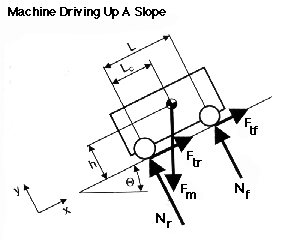Fm = mg (gravitational force of the machine) Nf (normal force of both front wheels) Nr (normal force of both rear wheels) Ftf = Nf µ (tractional force of both front wheels, caused by static friction) Ftr = Nr µ (tractional force of both rear wheels, caused by static friction) L (distance between the front and rear wheels) Lc (distance between the rear wheels and the center of mass) h (height of the center of mass) Ø (angle of incline plane)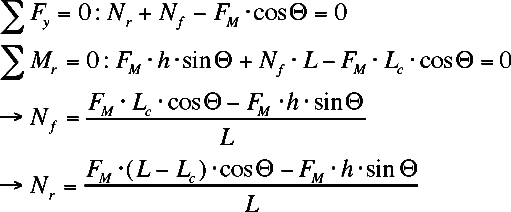Tipping of the Machine occurs at h=hmax when Nf=0: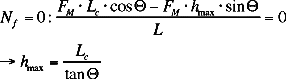What can be done to prevent the machine from tipping?

• moving the center of gravity farther from the rear wheels
• decreasing the slope angle

Four Wheel Drive:

The necessary static coefficient of friction (µ4WD) for a four wheel drive vehicle: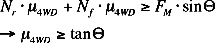What can be changed to allow for a lower coefficient of friction?

• Decrease the slope of the angle

Front Wheel Drive:

The necessary static coefficient of friction (µFWD) for a front wheel drive vehicle: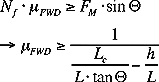What can be changed to allow for a lower coefficient of friction?

• decrease the anlge of the incline
• move the center of gravity closer to the front wheels
• shortening the distance between the front and rear wheels
• lowering the height of the center of gravity

Rear Wheel Drive:

The necessary static coefficient of friction (µRWD) for a rear wheel drive vehicle: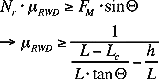What can be changed to allow for a lower coefficient of friction?

• decrease the angle of the incline
• move the center of gravity closer to the rear wheels
• increasing the height of the center of gravity

### A Wheel Driving Up a Step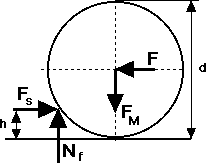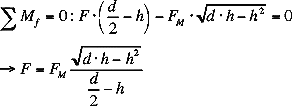0 < h < d/2